, 赵乃峰1, 王浩2, 张志超1,3
1. 清华大学 土木工程系, 北京 100084, 中国;
2. 新加坡南洋理工大学 土木与环境工程学院, 新加坡 639798, 新加坡;
3. 重庆大学 土木工程学院, 重庆 400045, 中国

Tsinghua thermodynamic soil model for simulating energy engineering projects
CHENG Xiaohui1, ZHAO Naifeng1, WANG Hao2, ZHANG Zhichao1,3
1. Department of Civil Engineering, Tsinghua University, Beijing 100084, China;
2. School of Civil and Environmental Engineering, Nanyang Technological University, Singapore 639798, Singapore;
3. School of Civil Engineering, Chongqing University, Chongqing 400045, China
Abstract: Energy piles utilize pile foundations to exchange heat with the surrounding rock and soil to facilitate efficient use of shallow geothermal energy supplies. An energy pile design is given that improves the thermo-mechanical coupling of the soil with the energy pile. Specifically, this paper summaries the influences of temperature and stress on the volume change of saturated clays with evaluations of the thermo-mechanical constitutive models in the literature. A Tsinghua thermodynamic soil (TTS) model is then used in finite element simulations of the thermo-mechanical behavior of elevated temperature oedometer tests of Kaolin clay with an energy pile. The predictions show that heating reduces the bearing capacity of the energy pile foundation, the interfacial normal stress and the shear stress. The results also show that the interfacial shear stress increases with increasing temperature and foundation depth, while the interfacial normal stress changes little. Cooling reduces the energy pile-soil interface interactions, increases foundation settling and reduces the bearing capacity.
Key words: energy underground structure    thermal creep test    non-equilibrium thermodynamics    thermo-mechanical coupling    constitutive model

1 饱和黏土热力耦合的体变规律与本构模拟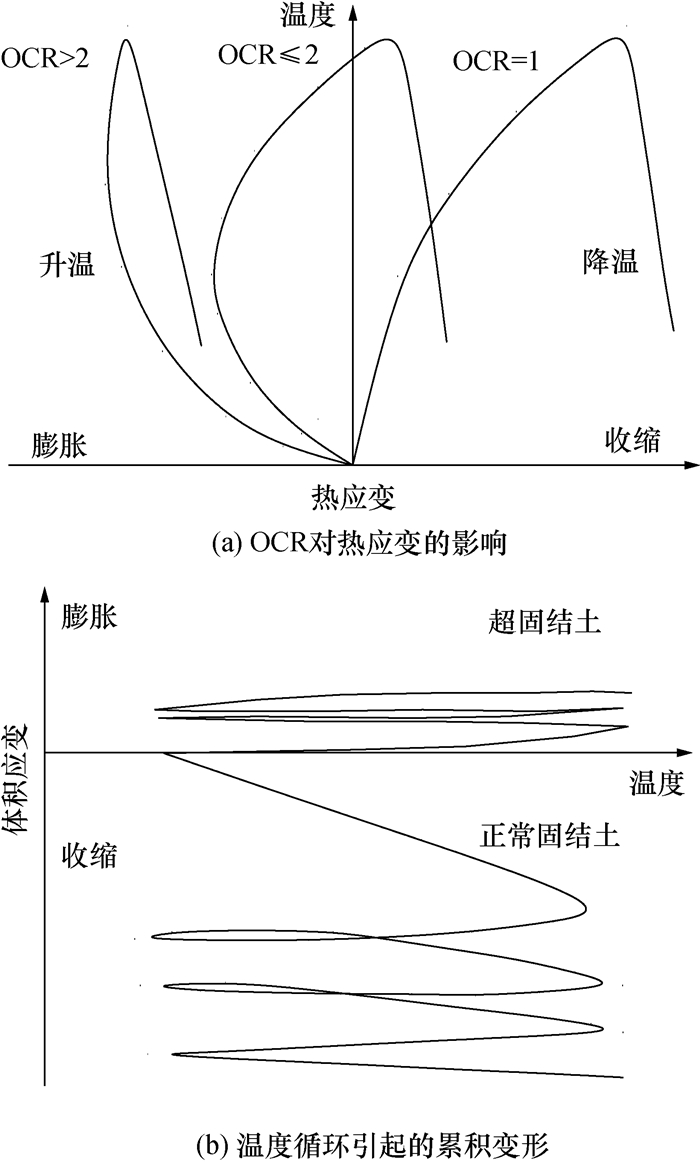图 1 饱和黏土的热应变发展规律

1) 正常固结土在升温过程中均发生体积收缩，温度体应变关系曲线具有非线性，此后的降温过程仍发生体积收缩，温度体应变关系曲线近似线性。

2) 轻微超固结土升温体积先膨胀后收缩，降温体积收缩；重超固结土升温体积膨胀，超固结比(overconsolidation ratio, OCR)越大，体积从膨胀转变到收缩的温度也越高。

3) 正常固结土多次温度循环过程中有显著的累积体缩变形产生，而超固结土温度循环后累积体积膨胀变形，累积的体缩/体胀变形随循环次数的增加而减少，多次循环后累积变形基本稳定。

2 TTS模型

TTS模型是以非平衡态热力学为基础的新一代岩土本构模型，能够为岩土材料力学响应提供更本质的物理基础。较弹塑性本构模型而言，TTS模型摒弃了经典塑性力学中的若干概念(屈服函数、流动法则、硬化规律等)，以颗粒固体流体动力学(granular solid hydrodynamics, GSH)为理论框架，能够统一描述岩土材料温度效应、临界状态、率相关性、各向异性、循环滞回、多场耦合、小应变刚度等重要性质[27-29]。限于篇幅，本文仅给出温度-应力耦合的TTS模型框架。

2.1 理论假定

TTS模型主要有3点理论假定：

1) 假设饱和多孔介质由固相和液相组成，液相又由结合水及自由水组成。随着温度的变化，结合水与自由水之间存在质量交换

2) 假设各相在空间上均为连续分布，整体方程则可表示为各相方程的叠加。

3) 假设不同相的温度相同，液相不产生固化和汽化。

2.2 弹性势能与有效应力

TTS模型采用超弹性理论来描述岩土材料的非线性弹性行为。在超弹性理论中，材料的应力应变关系通过自由能函数(弹性势能函数或应变余能函数)得到，完全满足热力学基本定律。为了合理描述岩土材料压硬性、压剪耦合与非等向固结剪切刚度，TTS模型的弹性势能函数为

 $\omega_{\mathrm{e}}=B\left(\varepsilon_{\mathrm{v}}^{\mathrm{e}}+c\right)^{n}\left[\left(\varepsilon_{\mathrm{v}}^{\mathrm{e}}\right)^{2}+\xi\left(\varepsilon_{\mathrm{s}}^{\mathrm{e}}\right)^{2}\right].$ (1)

 $\omega_{\mathrm{e}}=B\left(\varepsilon_{\mathrm{v}}^{\mathrm{e}}+c\right)^{n}\left[\left(\varepsilon_{\mathrm{v}}^{\mathrm{e}}\right)^{2}+\xi\left(\varepsilon_{\mathrm{s}}^{\mathrm{e}}\right)^{2}\right]+\int K \beta_{\mathrm{T}} \Delta T \mathrm{d} \varepsilon_{\mathrm{v}}^{\mathrm{e}}.$ (2)

 $\begin{array}{c} \sigma_{i j}^{\prime}=B\left(\varepsilon_{\mathrm{v}}^{\mathrm{e}}+c\right)^{n-1}\left[(n+2)\left(\varepsilon_{\mathrm{v}}^{\mathrm{e}}\right)^{2}+2 c \varepsilon_{\mathrm{v}}^{\mathrm{e}}+\right. \\ \left.n \xi\left(\varepsilon_{\mathrm{s}}^{\mathrm{e}}\right)^{2}\right] \delta_{i j}+K \beta_{\mathrm{T}} \Delta T \delta_{i j}+2 B \xi\left(\varepsilon_{\mathrm{v}}^{\mathrm{e}}+c\right)^{n} \varepsilon_{i j}^{\mathrm{e}}. \end{array}$ (3)

2.3 非弹性与能量耗散

1) 颗粒熵增方程。

 $\begin{array}{c} \rho_{\mathrm{s}} \dot{T}_{\mathrm{g}}^{\prime}=m_{2} m_{4} \dot{e}_{i j} \dot{e}_{i j}+m_{3} \dot{\varepsilon}_{\mathrm{v}}^{2}+ \\ \frac{m_{5} \pi_{k k} \alpha_{\mathrm{bf}} \phi_{\mathrm{b}}}{3 \phi_{\mathrm{s}}} \dot{T}-m_{4} T_{\mathrm{g}}^{\prime}. \end{array}$ (4)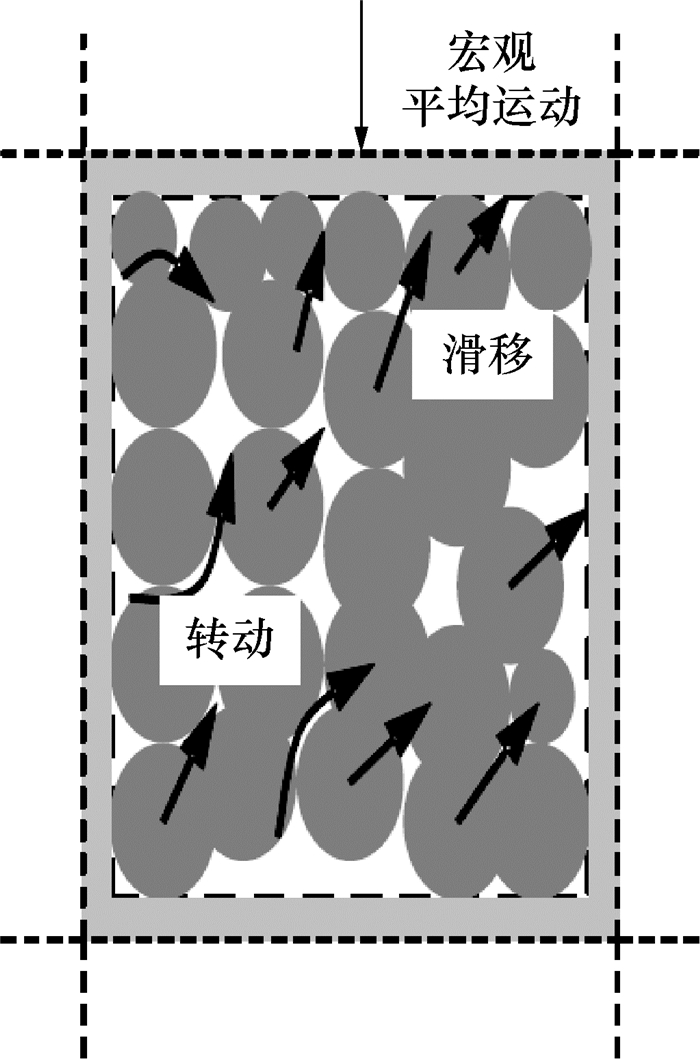图 2 颗粒涨落运动示意图

2) 弹性弛豫方程。

 $\dot{\varepsilon}_{i j}^{\mathrm{e}}=\dot{\varepsilon}_{i j}-\left(m_{1} T_{\mathrm{g}}^{\prime} \alpha_{\varepsilon}^{\mathrm{e}} \delta_{i j}+T_{\mathrm{g}}^{\prime} \alpha_{i j}^{\mathrm{e}}\right).$ (5)

2.4 模型参数

TTS模型参数包括超弹性参数4个、能量耗散参数或迁移系数4个、温度相关参数4个，共12个，如表 1所示。

 类别代码 取值 B0 2.35 B1 0.043 8 c 0.023 8 ξ 0.444 m1 0.333 m2 3.96 m3 0.43 m4 110 βs 0.054 6 αbf 0.035 7 kg 0.072 m5 5×10-9

1) 参数nBcξ

2) 迁移系数相关参数m1m2m3m4

3) 温度相关参数βsαbfkgm5

3 基于TTS模型的有限元分析 3.1 高岭土升温排水试验有限元分析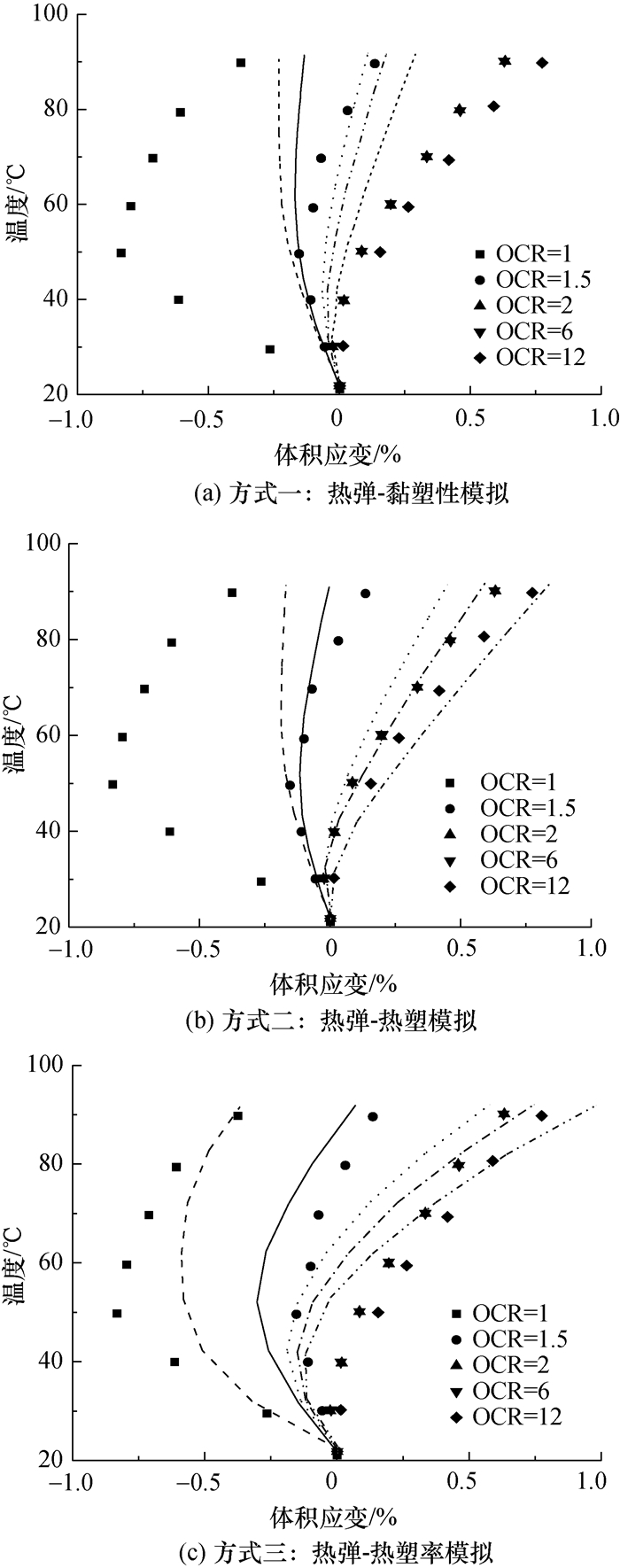图 3 高岭土热应变的有限元计算结果

3.2 能源结构温度-应力耦合平面应变有限元分析图 4 能源基础平面应变有限元模型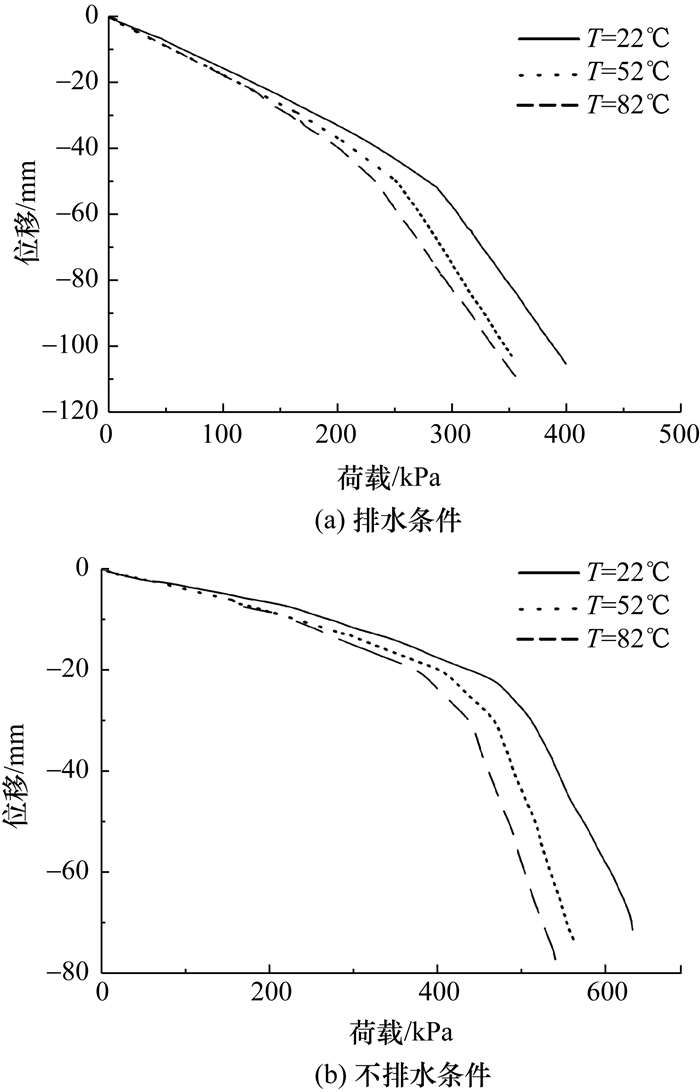图 5 不同温度的荷载位移曲线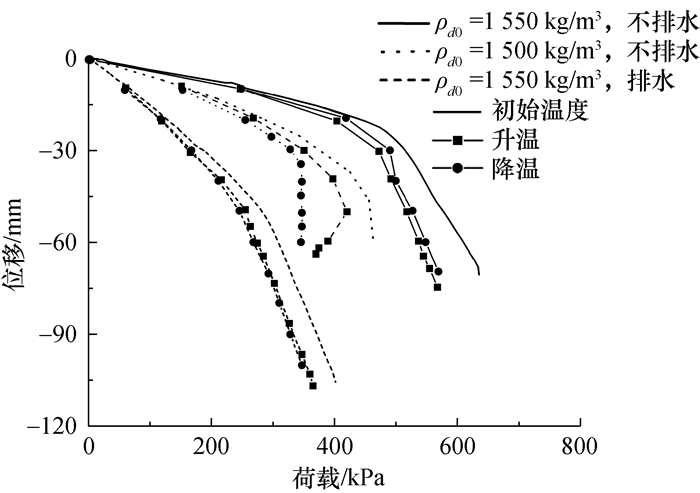图 6 温度循环的荷载位移曲线

4 结论

1) 饱和黏土的热应变与OCR有关，正常固结土升温降温过程均发生体积收缩；超固结土升温膨胀降温收缩；累积变形随循环次数的增加而减少，多次循环后累积变形基本稳定。热塑性本构模型能够较好描述黏土的热力特征，因本构模型的复杂性，尚未开展相关的有限元分析工作。

2) TTS模型是热弹-热塑率相关模型，可以反映岩土材料热胀冷缩的孔压增长与主固结、温度蠕变和热塑率相关性。模型特定参数8~10个。

3) 基于TTS模型，在给定参数条件下，在升温过程中，能源基础的承载能力有微小降低。界面正应力、切应力均发生衰减，随着基础深度的增大，界面切应力随温度升高呈非线性增加，而界面正应力变化较小。升温降温后，桩土界面的接触减弱，基础沉降有所增大、承载能力有一定降低。

4) 现有模拟中，仍以热弹耦合的本构模型为主，有必要进一步开展以热塑耦合本构模型为基础的有限元模拟；值得注意的是，现有热塑耦合本构模型没有考虑温度蠕变的影响，开展热弹-热黏塑性本构模型研究也十分重要。

  桂树强, 程晓辉. 能源桩换热过程中结构响应原位试验研究[J]. 岩土工程学报, 2014, 36(6): 1087-1094. GUI S Q, CHENG X H. In-situ tests on structural responses of energy piles during heat exchanging process[J]. Chinese Journal of Geotechnical Engineering, 2014, 36(6): 1087-1094. (in Chinese)  LALOUI L, DI DONNA A. Energy geostructures:Innovation in underground engineering[M]. London: ISTE, 2013.  AMIS T, ROBINSON C A W, WONG S. Integrating geothermal loops into the diaphragm walls of the Knightsbridge Palace Hotel project[C]//Proceedings of the 11th DFI/EFFC International Conference on Geotechnical Challenges in Urban Regeneration. HAWTHOME N J, KENT M I. Deep Foundation Institute. London, Britain: European Federation of Foundation Contractors, 2010.  FRANZIUS J N, PRALLE N. Turning segmental tunnels into sources of renewable energy[J]. Proceedings of the Institution of Civil Engineers-Civil Engineering, 2011, 164(1): 35-40.  FRODL S, FRANZIUS J N, BARTL T. Design and construction of the tunnel geothermal system in Jenbach[J]. Geomechanics and Tunnelling, 2010, 3(5): 658-668.  刘汉龙, 孔纲强, 吴宏伟. 能量桩工程应用研究进展及PCC能量桩技术开发[J]. 岩土工程学报, 2014, 36(1): 176-181. LIU H L, KONG G Q, WU H W. Applications of energy piles and technical development of PCC energy piles[J]. Chinese Journal of Geotechnical Engineering, 2014, 36(1): 176-181. (in Chinese)  江强强, 焦玉勇, 骆进, 等. 能源桩传热与承载特性研究现状及展望[J]. 岩土力学, 2019, 40(9): 3351-3362, 3372. JIANG Q Q, JIAO Y Y, LUO J, et al. Review and prospect on heat transfer and bearing performance of energy piles[J]. Rock and Soil Mechanics, 2019, 40(9): 3351-3362, 3372. (in Chinese)  Ground Source Heat Pump Association (GSHPA). Thermal pile design, installation & materials standards[M]. Milton Keynes, UK: National Energy Centre, 2013.  PARRIAUX A, TACHER L. Utilisation de la chaleur du sol par des ouvrages de fondation et de soutènement en béton. In Guide Pour la Conception, la Réalisation et la Maintenance[R]. Zurich, Switzerland: Architeets, 2005.  中华人民共和国住房与城乡建设部.桩基地热能利用技术标准: JGJ/T 438-2018[S].北京: 中国建筑工业出版社, 2018. Ministry of Housing and Urban-Rural Development of the People's Republic of China. Technical standard for utilization of geothermal energy through piles: JGJ/T 438-2018[S]. Beijing: China Architecture & Building Press, 2018. (in Chinese)  ABUEL-NAGA H M, BERGADO D T, BOUAZZA A, et al. Volume change behaviour of saturated clays under drained heating conditions:Experimental results and constitutive modeling[J]. Canadian Geotechnical Journal, 2007, 44(8): 942-956.  DI DONNA A, LALOUI L. Response of soil subjected to thermal cyclic loading:Experimental and constitutive study[J]. Engineering Geology, 2015, 190: 65-76.  SULTAN N, DELAGE P, CUI Y J. Temperature effects on the volume change behaviour of Boom clay[J]. Engineering Geology, 2002, 64(2-3): 135-145.  MONFARED M, SULEM J, DELAGE P, et al. A laboratory investigation on thermal properties of the opalinus claystone[J]. Rock Mechanics and Rock Engineering, 2011, 44(6): 735.  白冰, 赵成刚. 温度对粘性土介质力学特性的影响[J]. 岩土力学, 2003, 24(4): 533-537. BAI B, ZHAO C G. Temperature effects on mechanical characteristics of clay soils[J]. Rock and Soil Mechanics, 2003, 24(4): 533-537. (in Chinese)  费康, 戴迪, 付长郓. 热-力耦合作用下黏性土体积变形特性试验研究[J]. 岩土工程学报, 2019, 41(9): 1752-1758. FEI K, DAI D, FU C Y. Experimental study on volume change behavior of clay subjected to thermo-mechanical loads[J]. Chinese Journal of Geotechnical Engineering, 2019, 41(9): 1752-1758. (in Chinese)  PUSCH R. Permanent crystal lattice contraction, a primary mechanism in thermally induced alteration of Na bentonite[J]. MRS Online Proceedings Library, 1986, 84: 791-802.  LALOUI L. Thermo-mechanical behaviour of soils[J]. Revue Francaise de Génie Civil, 2001, 5(6): 809-843.  ZHANG S, LENG W M, ZHANG F, et al. A simple thermo-elastoplastic model for geomaterials[J]. International Journal of Plasticity, 2012, 34: 93-113.  XIONG Y L, ZHANG S, YE G L, et al. Modification of thermo-elasto-viscoplastic model for soft rock and its application to THM analysis of heating tests[J]. Soils and Foundations, 2014, 54(2): 176-196.  CUI Y J, SULTAN N, DELAGE P. A thermomechanical model for saturated clays[J]. Canadian Geotechnical Journal, 2000, 37(3): 607-620.  LALOUI L, FRANCOIS B. ACMEG-T:Soil thermoplasticity model[J]. Journal of Engineering Mechanics, 2009, 135(9): 932-944.  姚仰平, 杨一帆, 牛雷. 考虑温度影响的UH模型[J]. 中国科学:技术科学, 2011, 54(2): 158-169. YAO Y P, YANG Y F, NIU L. UH model considering temperature effect[J]. Science China Technological Sciences, 2011, 54(1): 190-202. (in Chinese)  DI DONNA A, LORIA A F R, LALOUI L. Numerical study of the response of a group of energy piles under different combinations of thermo-mechanical loads[J]. Computers and Geotechnics, 2016, 72: 126-142.  SURYATRIYASTUTI M E, MROUEH H, BURLON S. Understanding the temperature-induced mechanical behaviour of energy pile foundations[J]. Renewable and Sustainable Energy Reviews, 2012, 16(5): 3344-3354.  LORIA A F R, LALOUI L. Thermally induced group effects among energy piles[J]. Géotechnique, 2017, 67(5): 374-393. DOI:10.1680/jgeot.16.P.039  ZHANG Z, CHENG X H. A fully coupled THM model based on a non-equilibrium thermodynamic approach and its application[J]. International Journal for Numerical and Analytical Methods in Geomechanics, 2017, 41(4): 527-554.  ZHANG Z C, CHENG X H. A thermodynamic constitutive model for undrained monotonic and cyclic shear behavior of saturated soils[J]. Acta Geotechnica, 2015, 10(6): 781-796.  ZHANG Z C, CHENG X H. A thermo-mechanical coupled constitutive model for clay based on extended granular solid hydrodynamics[J]. Computers and Geotechnics, 2016, 80: 373-382.  邵玉娴.粘性土工程性质的温度效应试验研究[D].南京: 南京大学, 2011. SHAO Y X. Experimental study on temperature effect on engineering properties of clayey soils[D]. Nanjing: Nanjing University, 2011. (in Chinese)  JIANG Y M, LIU M. Granular solid hydrodynamics[J]. Granular Matter, 2009, 11(3): 139-156. DOI:10.1007/s10035-009-0137-3  CEKEREVAC C, LALOUI L, VULLIET L. A novel triaxial apparatus for thermo-mechanical testing of soils[J]. Geotechnical Testing Journal, 2005, 28(2): 161-170.